# BLACK HOLES and WORMHOLES PRODUCTION AT THE LHC

• Slides: 20
Download presentationBLACK HOLES and WORMHOLES PRODUCTION AT THE LHC I. Ya. Aref’eva Steklov Mathematical Institute, Moscow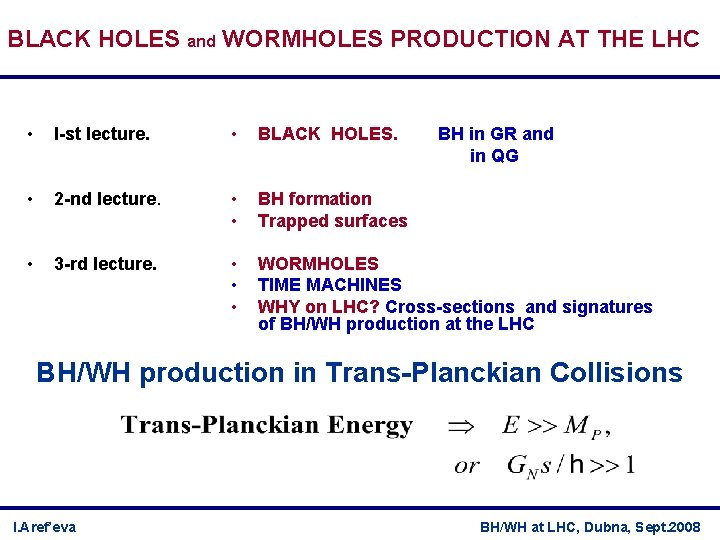BLACK HOLES and WORMHOLES PRODUCTION AT THE LHC • I-st lecture. • BLACK HOLES. • 2 -nd lecture. • • BH formation Trapped surfaces • 3 -rd lecture. • • • WORMHOLES TIME MACHINES WHY on LHC? Cross-sections and signatures of BH/WH production at the LHC BH in GR and in QG BH/WH production in Trans-Planckian Collisions I. Aref’eva BH/WH at LHC, Dubna, Sept. 2008I-st lecture. Outlook: • BLACK HOLES in GR • Historical Remarks • SCHWARZSCHILD BH • Event Horizon • Trapped Surfaces • BH Formation Refs. : L. D. Landau, E. M. Lifshitz, The Classical Theory of Fields, II v. Hawking S. , Ellis J. The large scale structure of space-time. R. Wald, General Relativity, 1984 S. Carroll, Spacetime and Geometry. An introduction to general relativity, 2004 • BLACK HOLES in QG • BLACK HOLES in Semi-classical approximation to QG I. Aref’eva BH/WH at LHC, Dubna, Sept. 2008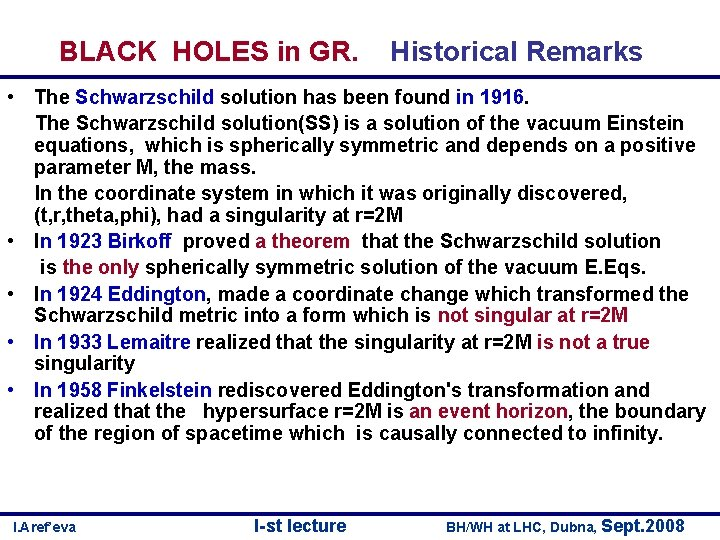BLACK HOLES in GR. Historical Remarks • The Schwarzschild solution has been found in 1916. The Schwarzschild solution(SS) is a solution of the vacuum Einstein equations, which is spherically symmetric and depends on a positive parameter M, the mass. In the coordinate system in which it was originally discovered, (t, r, theta, phi), had a singularity at r=2 M • In 1923 Birkoff proved a theorem that the Schwarzschild solution is the only spherically symmetric solution of the vacuum E. Eqs. • In 1924 Eddington, made a coordinate change which transformed the Schwarzschild metric into a form which is not singular at r=2 M • In 1933 Lemaitre realized that the singularity at r=2 M is not a true singularity • In 1958 Finkelstein rediscovered Eddington's transformation and realized that the hypersurface r=2 M is an event horizon, the boundary of the region of spacetime which is causally connected to infinity. I. Aref’eva I-st lecture BH/WH at LHC, Dubna, Sept. 2008BLACK HOLES in GR. Historical Remarks • In 1950 Synge constructed a systems of coordinates that covers the complete analytic extension of the SS • In 1960 Kruskal and Szekeres (independently) discovered a single most convenient system that covers the complete analytic extension of SS • In 1964 Penrose introduced the concept of null infinity, which made possible the precise general definition of a future event horizon as the boundary of the causal past of future null infinity. • In 1965 Penrose introduced the concept of a closed trapped surface and proved the first singularity theorem (incompleteness theorem). • Hawking-Penrose theorem: a spacetime with a complete future null infnity which contains a closed trapped surface must contain a future event horizon (H-E-books, 9 -2 -1) I. Aref’eva I-st lecture BH/WH at LHC, Dubna, Sept. 2008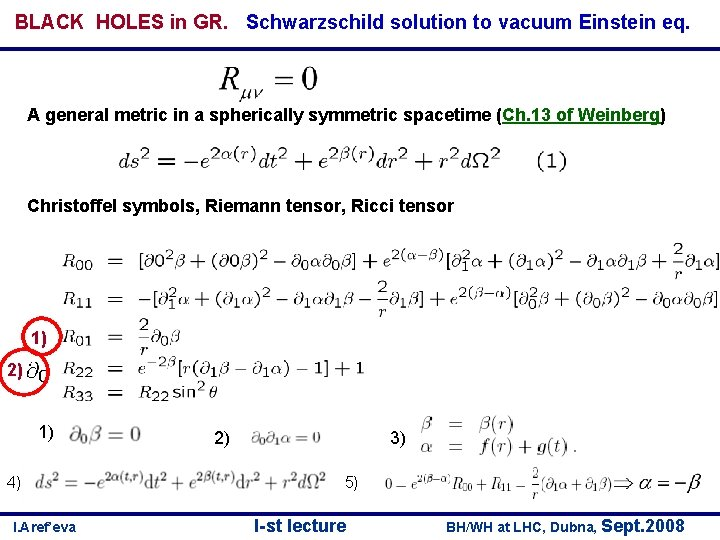BLACK HOLES in GR. Schwarzschild solution to vacuum Einstein eq. A general metric in a spherically symmetric spacetime (Ch. 13 of Weinberg) Christoffel symbols, Riemann tensor, Ricci tensor 1) 2) 1) 4) I. Aref’eva 3) 2) 5) I-st lecture BH/WH at LHC, Dubna, Sept. 2008BLACK HOLES in GR. Schwarzschild solution to vacuum Einstein eq. A general metric in a spherically symmetric spacetime (Ch. 13 of Weinberg) Christoffel symbols, Riemann tensor, Ricci tensor I. Aref’eva I-st lecture BH/WH at LHC, Dubna, Sept. 2008BLACK HOLES in GR. Schwarzschild solution • Asymptoticaly flat • Birkhoff's theorem: Schwarzschild solution is the unique spherically symmetric vacuum solution • Singularity I. Aref’eva I-st lecture BH/WH at LHC, Dubna, Sept. 2008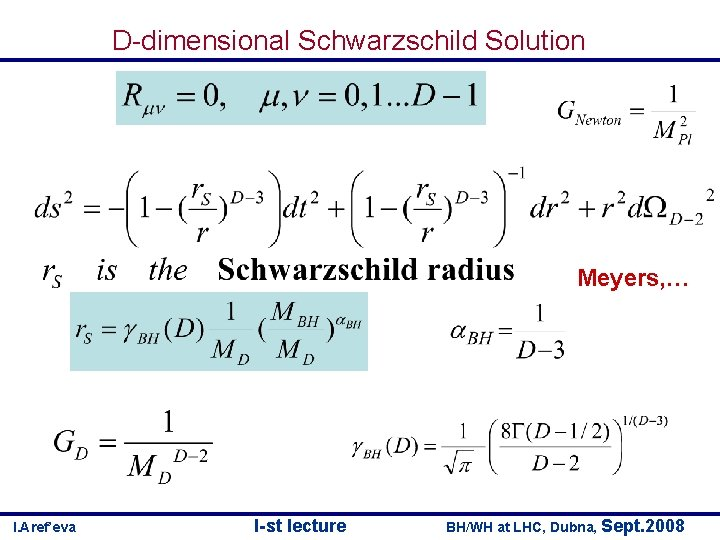D-dimensional Schwarzschild Solution Meyers, … I. Aref’eva I-st lecture BH/WH at LHC, Dubna, Sept. 2008BLACK HOLES in GR. Schwarzschild solution • Geodesics Null Geodesics I. Aref’eva I-st lecture BH/WH at LHC, Dubna, Sept. 2008BLACK HOLES in GR. Schwarzschild solution Null Geodesics Regge-Wheeler coordinates I. Aref’eva I-st lecture BH/WH at LHC, Dubna, Sept. 2008BLACK HOLES in GR. Schwarzschild solution Eddington-Finkelstein coordinates The determinant of the metric is is regular at r=2 GM r r=0 I. Aref’eva r=2 GM I-st lecture BH/WH at LHC, Dubna, Sept. 2008BLACK HOLES in GR. Schwarzschild solution Kruskal coordinates Kruskal diagram I. Aref’eva I-st lecture BH/WH at LHC, Dubna, Sept. 2008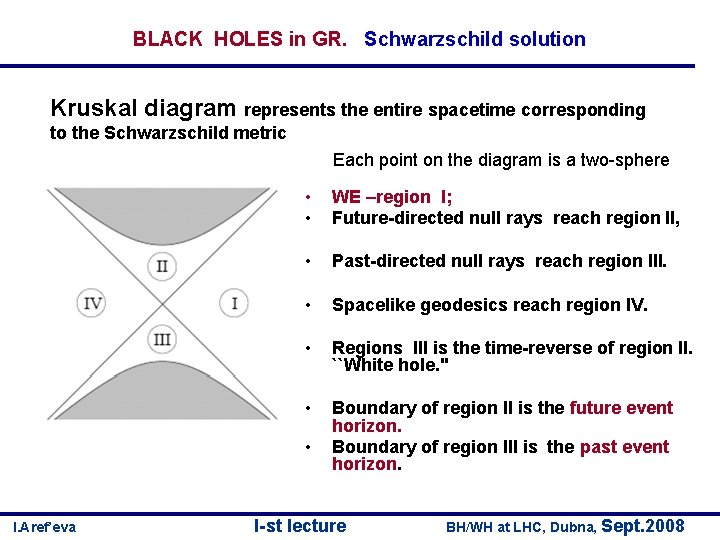BLACK HOLES in GR. Schwarzschild solution Kruskal diagram represents the entire spacetime corresponding to the Schwarzschild metric Each point on the diagram is a two-sphere • • WE –region I; Future-directed null rays reach region II, • Past-directed null rays reach region III. • Spacelike geodesics reach region IV. • Regions III is the time-reverse of region II. ``White hole. '' • Boundary of region II is the future event horizon. Boundary of region III is the past event horizon. • I. Aref’eva I-st lecture BH/WH at LHC, Dubna, Sept. 2008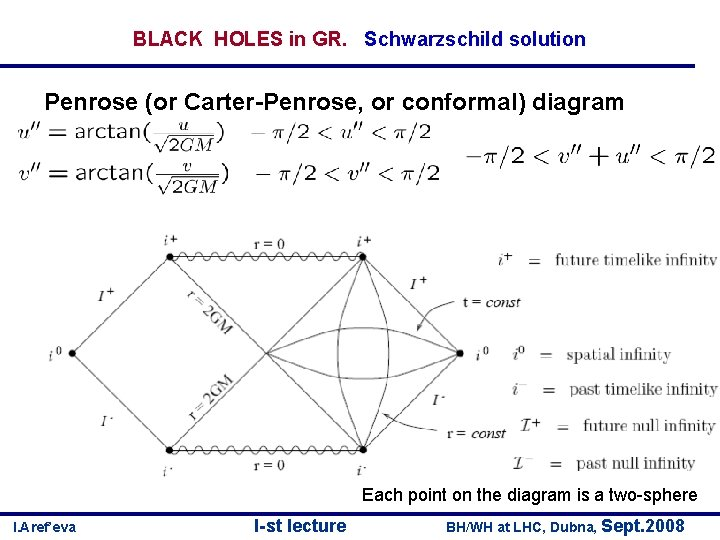BLACK HOLES in GR. Schwarzschild solution Penrose (or Carter-Penrose, or conformal) diagram Each point on the diagram is a two-sphere I. Aref’eva I-st lecture BH/WH at LHC, Dubna, Sept. 2008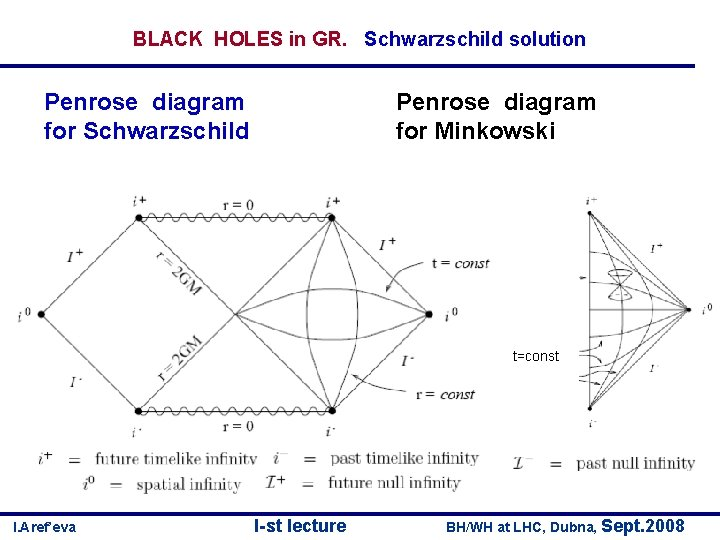BLACK HOLES in GR. Schwarzschild solution Penrose diagram for Schwarzschild Penrose diagram for Minkowski t=const I. Aref’eva I-st lecture BH/WH at LHC, Dubna, Sept. 2008Event Horizons The event horizon is always a null hypersurface. The event horizon does not depend on a choice of foliation. The event horizon always evolves continuously. The event horizon captures the intuitive idea of the boundary of what can reach observers at infinity. I. Aref’eva I-st lecture BH/WH at LHC, Dubna, Sept. 2008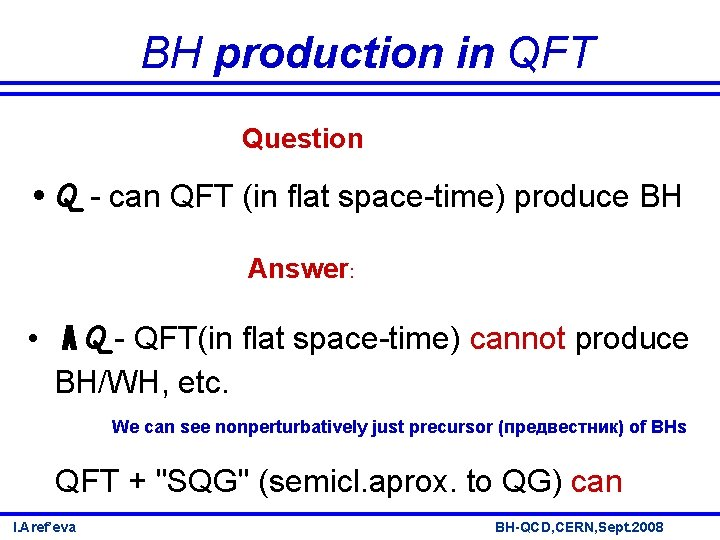BH production in QFT Question • Q - can QFT (in flat space-time) produce BH Answer: • A Q - QFT(in flat space-time) cannot produce BH/WH, etc. We can see nonperturbatively just precursor (предвестник) of BHs QFT + "SQG" (semicl. aprox. to QG) can I. Aref’eva BH-QCD, CERN, Sept. 2008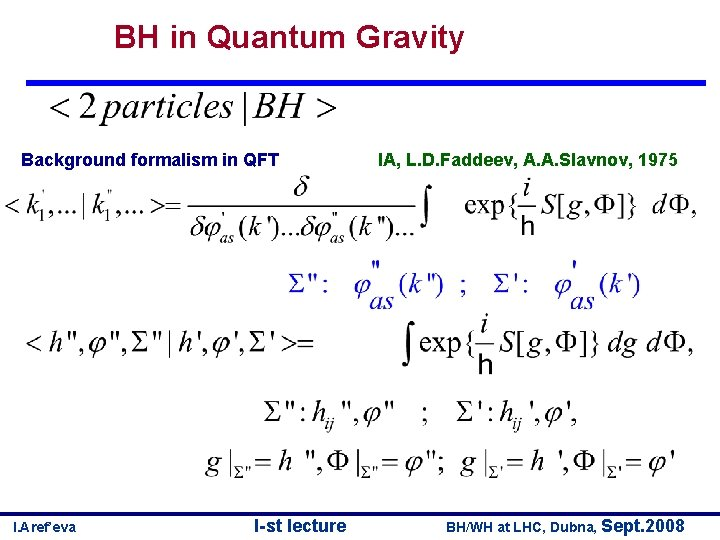BH in Quantum Gravity Background formalism in QFT I. Aref’eva I-st lecture IA, L. D. Faddeev, A. A. Slavnov, 1975 BH/WH at LHC, Dubna, Sept. 2008AQ. Analogy with Solitons How to see these solitons in QFT? • perturbative S-matrix for phi-particles - no indications of solitons • Exact nonperturbative S-matrix for phi-particles – extra poles - indications of a soliton-antisoliton state IA, V. Korepin, 1974 I. Aref’eva BH-QCD, CERN, Sept. 2008